Function Repository Resource:

# GridTableForm

Arrange the elements of the argument in a two-dimensional grid with row coloring that helps comprehension

Contributed by: Anton Antonov
 ResourceFunction["GridTableForm"][arg] arranges the elements of arg in a two-dimensional grid.

## Details and Options

ResourceFunction["GridTableForm"] behaves like TableForm but its implementation uses Grid.
If the first argument cannot be interpreted as a two-dimensional full array, it is completed to a two-dimensional array using empty strings.
If necessary, the rows are completed with empty strings.
ResourceFunction["GridTableForm"] takes all options of Grid, along with the following additional options:

## Examples

### Basic Examples (2)

Here is a grid table form of a full integer array:

 In:=Out=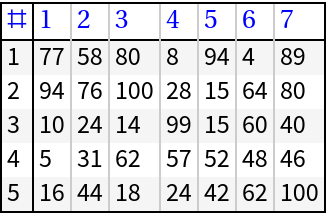Here is a grid table form of a nested list of integers that is not a full array:

 In:=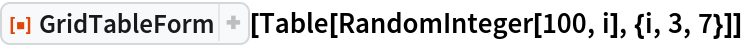Out=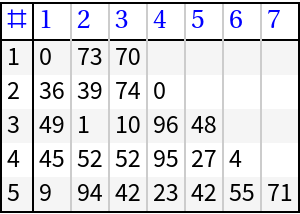### Scope (4)

 In:=Out=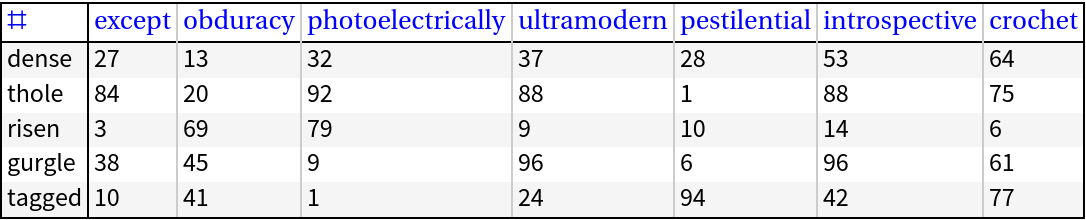If needed, various completions of the data and table headings are made in order to produce the final tabular form:

 In:=Out=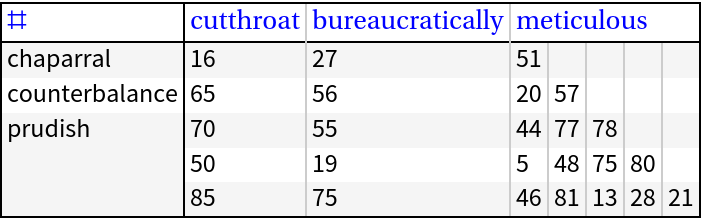GridTableForm can be invoked over Dataset objects; if the dataset has column names, then they are used as table headings:

 In:=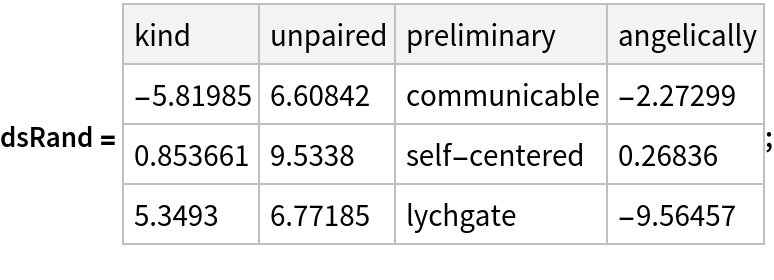In:=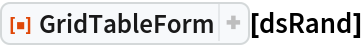Out=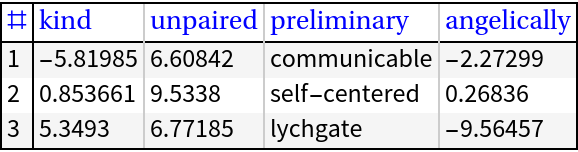In:=Out=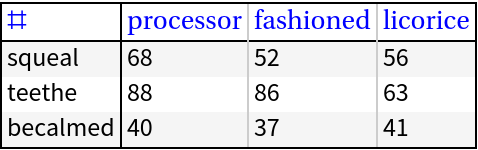### Options (4)

#### RowBackground (1)

The following table demonstrates the effect of different row background specifications:

 In:=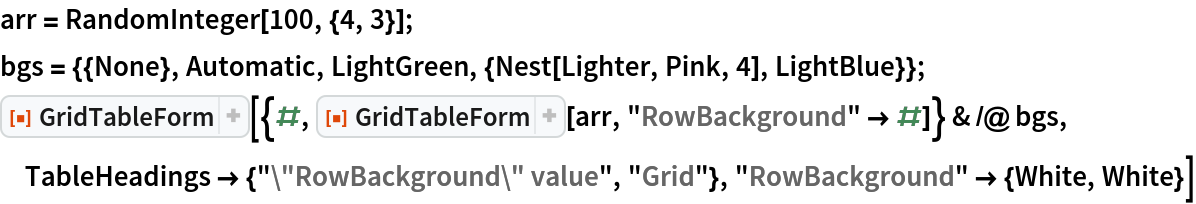Out=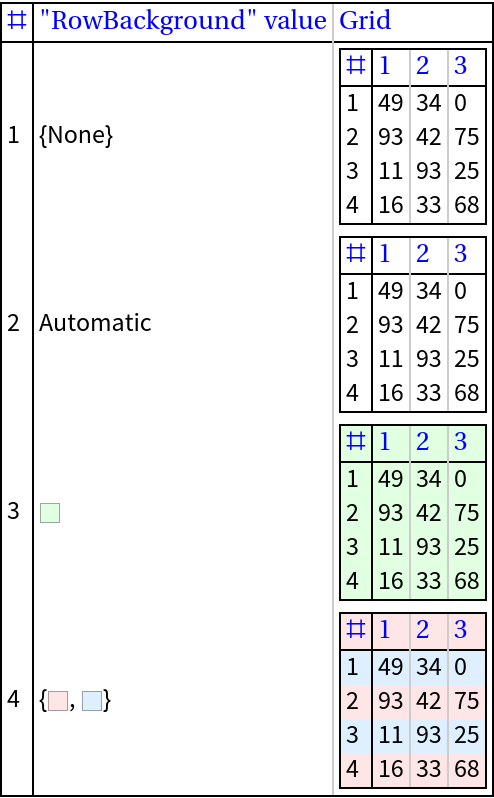The following table demonstrates the effect of different table headings specifications:

 In:=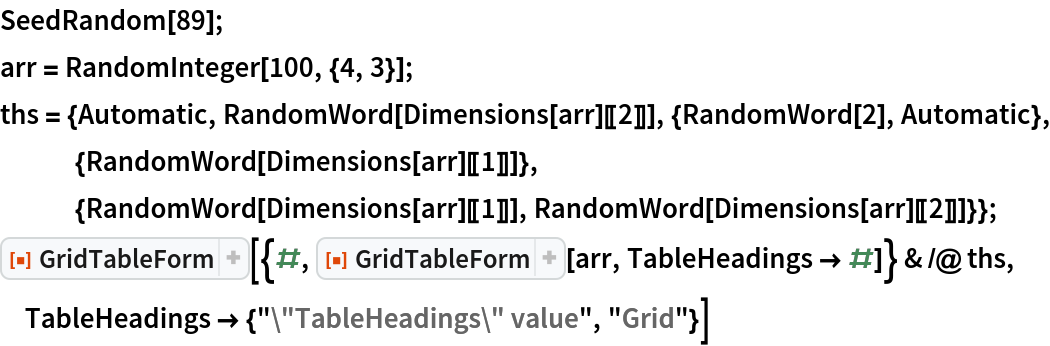Out=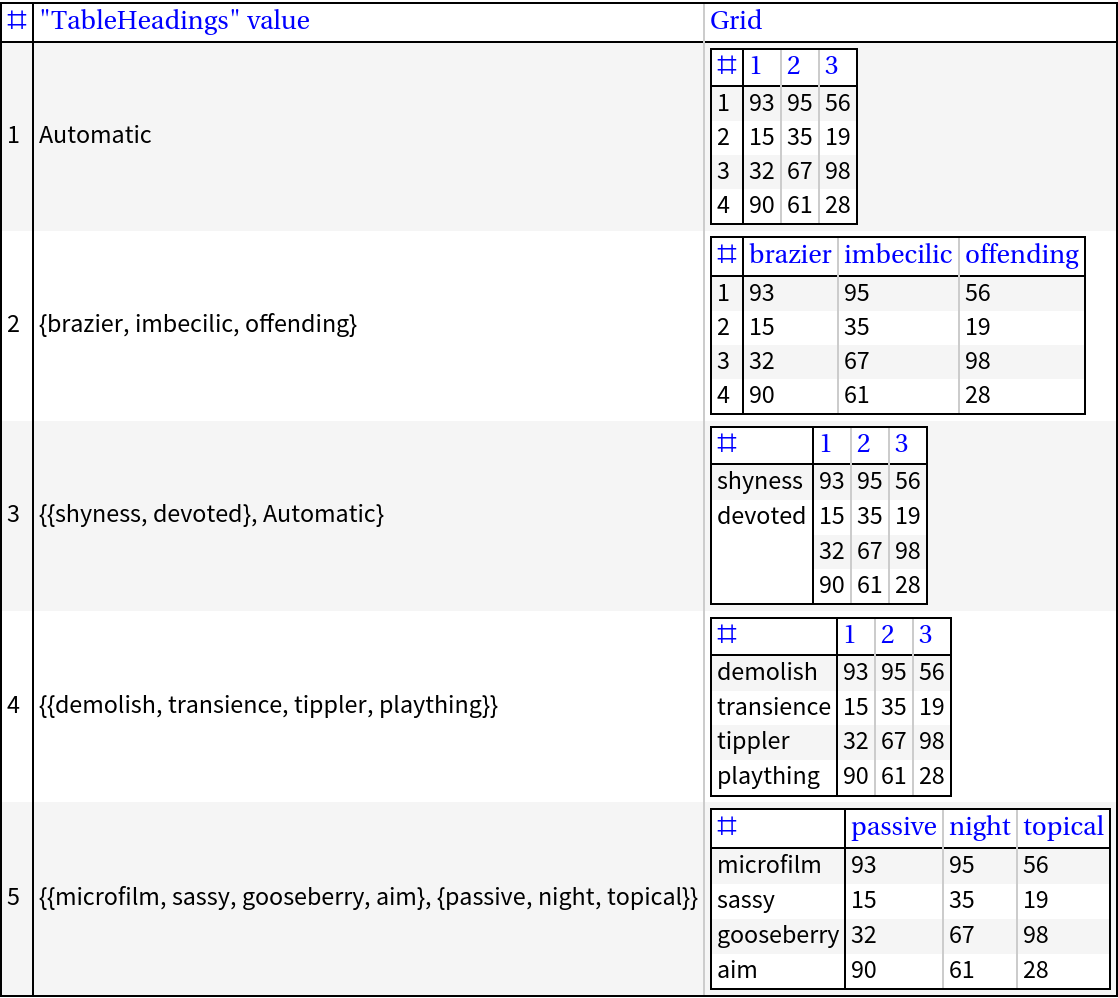If the row headings or column headings are too short, they are completed with SpanFromAbove and SpanFromLeft, respectively:

 In:=Out=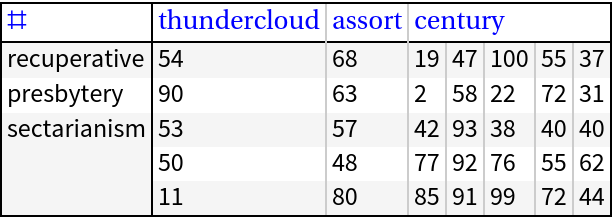#### Grid options (1)

GridTableForm takes all options of Grid:

 In:=Out=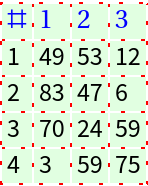### Applications (3)

Show a sample of machine learning data with corresponding variable names:

 In:=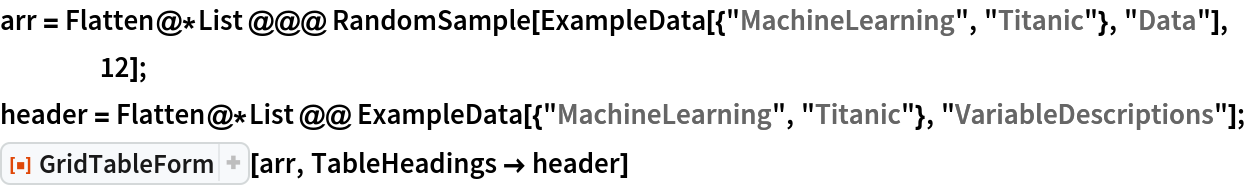Out=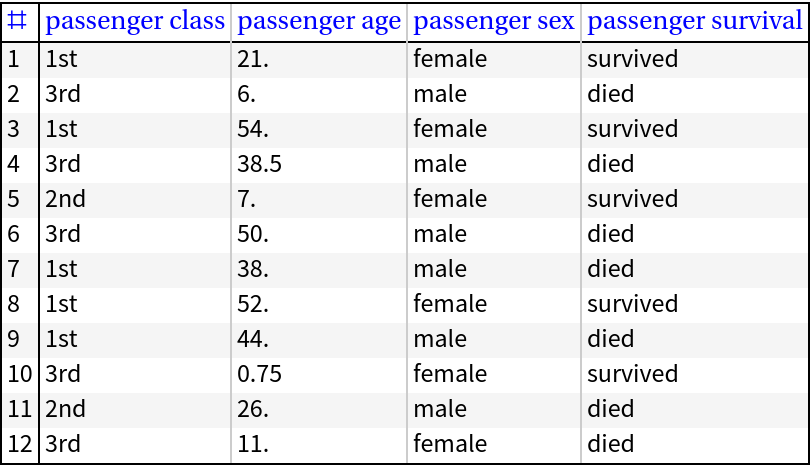When studying data arrays, the use of the ResourceFunction RecordsSummary can be completed with GridTableForm:

 In:=In:=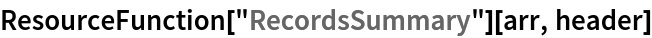Out=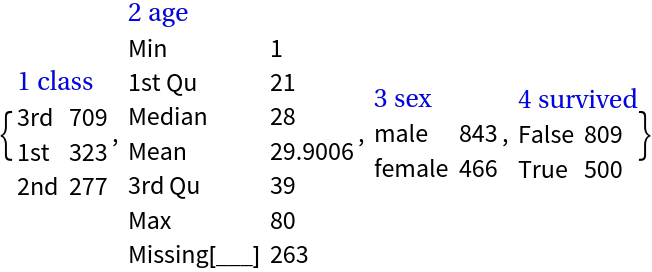In:=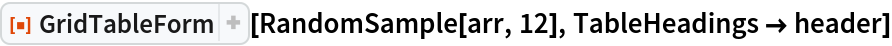Out=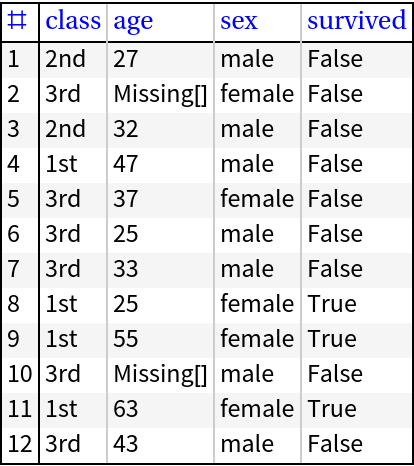Show all rows and columns of a dataset:

 In:=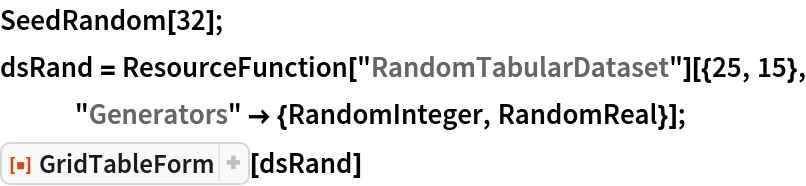Out=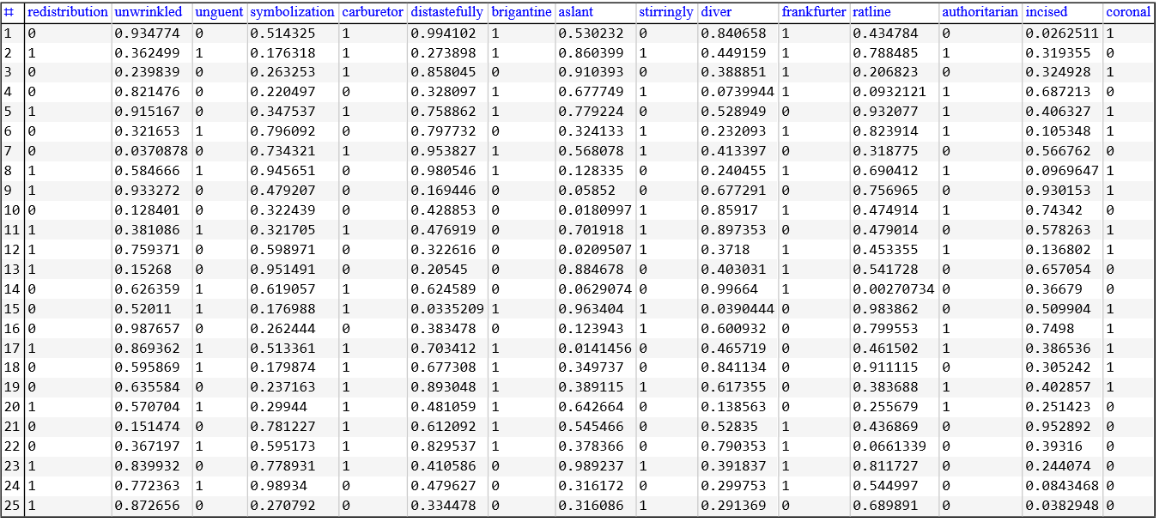### Properties and Relations (2)

GridTableForm is related to both TableForm and Grid. The relation with TableForm can be seen in this example:

 In:=In:=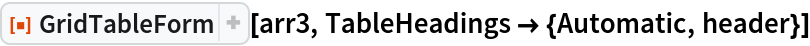Out=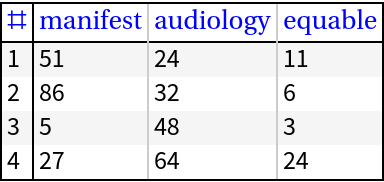In:=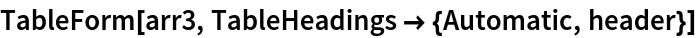Out=In order to produce a similar result using Grid, several data "augmentations" and option specifications have to be made:

 In:=Out=### Possible Issues (3)

If the specified table headings are styled, the specification given to the option "TableHeadingsStyle" has no effect:

 In:=Out=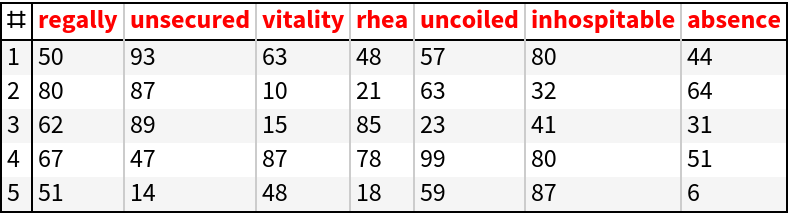The background is extended for the extended row names:

 In:=Out=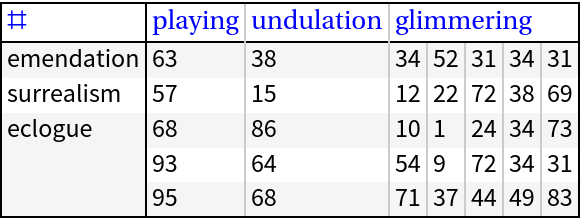If the specified table headings value is a list with one element, that element is treated as a row names specification. Compare the following examples:

 In:=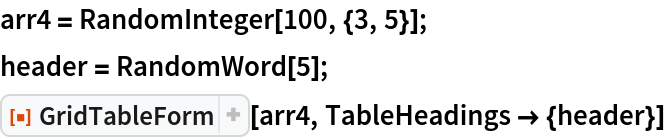Out=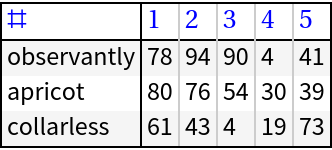In:=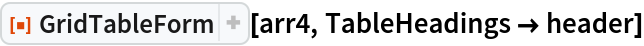Out=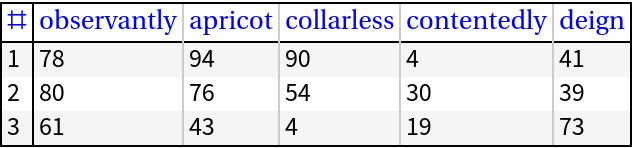### Neat Examples (1)

This example is similar to the "neat example" in the function page of TableForm:

 In:=Out=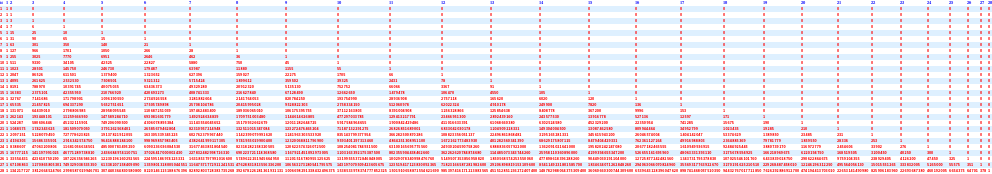Anton Antonov

## Version History

• 1.1.0 – 14 December 2021
• 1.0.0 – 03 January 2020